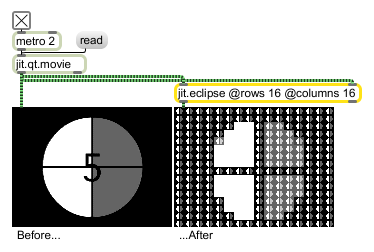# jit.eclipse

Meta-imaging

## Description

The jit.eclipse object performs meta-imaging on two 4-plane char input matrices.

A jit.eclipse object divides a matrix sent into the left inlet into a grid with a specified number of rows and columns. Each box in the grid contains a scaled-down representation of the overall matrix. Each box is then tinted so that the overall image resembles a second matrix (sent into the right inlet). If the same image is used in both inputs, the result is a self-similar (or meta-) image.

Additional features such as threshold inversion, scalar tinting, and a choice of additive or multiplicative tinting are supported. There are also four modes that switch between color and monochrome treatment of the two matrices.

## Matrix Operator

matrix inputs:2, matrix outputs:1
 Name IOProc Planelink Typelink Dimlink Plane Dim Type in2 resamp 1 1 1 4 1 char out n/a 1 1 1 4 1 char

## Attributes

Name Type g/s Description
blue float The amount of additional scaling applied to the blue channel (plane 3) of the output matrix (default = 0.)
columns int The number of columns in the meta-image (default = 1)
green float The amount of additional scaling applied to the green channel (plane 2) of the output matrix (default = 0.)
inv int Invert color flag (default = 0) When the flag is set, RGB attributes are inverted in regions of the output image that are inverted.
mode int Color mode by which the object tints the output matrix (default = 0 (both matrices color))
0 = both matrices are color
1 = matrix 1 is converted to greyscale
2 = matrix 2 is converted to greyscale
3 = both matrices are converted to greyscale
op int The mathematical operation used for tinting (default = 0 (addition))
1 = multiplication
red float The amount of additional scaling applied to the red channel (plane 1) of the output matrix (default = 0.)
rows int The number of rows in the meta-image (default = 1)
thresh float The threshold luminosity value around which grid regions in the meta-image are inverted in color (default = -1.01)
tint int Tint mode flag (default = 1) When the flag is set, tinting is based on the second input matrix.

## Examples